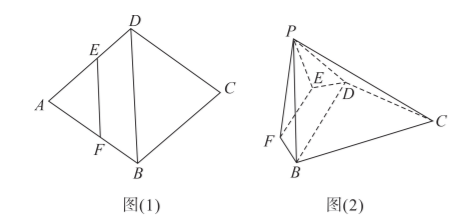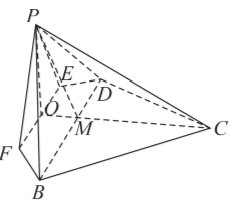(1) 当 $\lambda$ 为何值时, $B F \perp P D$;
(2) 若直线 $P C$ 与平面 $B C D E F$ 所成角的正切值为 $\frac{1}{3}$, 求平面 $P E F$ 和平面 $P B D$ 夹角的 大小.【答案】20.【解析】(1) $\because$ 菱形 $A B C D$ 中, $\angle A B C=$ $120^{\circ}$, 故 $\angle A=60^{\circ}$, $A B=A D$,
$\therefore \triangle A B D$ 是等边三 角形, 又 $\overrightarrow{E F}=\lambda \overrightarrow{D B}$,
$\therefore E F / / B D, \quad \therefore$
$\triangle P E F$ 也是等边三角形,
$\because$ 平面 $P E F \perp$ 平面 $B C D E F$, 取 $E F$ 的中点 $O$, 则 $P O \perp E F$, 且 $P O \perp$ 平面 $B C D E F$, 连接 $D O$, 由 $B F \perp P D$, 而 $P O \perp B F, D O \cap P O$, $\therefore B F \perp$ 平面 $P O D, \therefore B F \perp O D$, 延长 $D O$ 交 $A B$ 于点 $N$, 则 $D N \perp A B$, 又 $\because A O \perp B D, \therefore O$ 为 $\triangle A B D$ 的重心, 又 $O$ 点在 $E F$ 上, $E F / / B D, \therefore \overrightarrow{E F}=\frac{2}{3} \overrightarrow{D B}$, 即 $\lambda=\frac{2}{3}$.
(2) 方法一: 由 (1) 连接 $C O$, 设 $\triangle A B D$ 边长为 $a$, 则 $|P O|=\frac{\sqrt{3}}{2} \lambda a,|C O|=\frac{\sqrt{3}}{2}(2-\lambda) a$,
$\because P O \perp$ 平面 $B C D E F$,
$\therefore$ 直线 $P C$ 与平面 $B C D E F$ 所成角为 $\angle P C O$,

$\therefore \tan \angle P C O=\frac{|P O|}{|C O|}=\frac{\lambda}{2-\lambda}=\frac{1}{3}$, 解得 $\lambda=\frac{1}{2}$,
$\therefore E F$ 是 $\triangle A B D$ 的中位线,

$\because E F / / B D, E F \not \subset$ 平面 $P B D$,
$\therefore E F / /$ 平面 $P B D$,

$\therefore E F / / l$, 同理 $l / / B D$,

$\therefore E F \perp$ 平面 $P O M, \therefore l \perp$ 平面 $P O M$,
$\therefore \angle O P M$ 就是平面 $P E F$ 和平面 $P B D$ 所成二 面角的平面角,